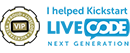## Arranging objects of equal width and height in 'matrix form'

Got a LiveCode personal license? Are you a beginner, hobbyist or educator that's new to LiveCode? This forum is the place to go for help getting started. Welcome!

Moderators: Klaus, FourthWorld, heatherlaine, kevinmiller

[-hh]
VIP Livecode Opensource BackerPosts: 2262
Joined: Thu Feb 28, 2013 11:52 pm
Location: Göttingen, DE

### Arranging objects of equal width and height in 'matrix form'

Because it's Sunday today here a utility script that may help some of you.

Code: Select all

``````-- Creates a list of rectangles for objects
-- of equal width and height arranged as a
-- 'matrix' (rows and columns).
-- nr = number of rows of the objects
-- nc = number of columns of the objects
-- w = the width of each object
-- h = the height of each object
-- l = the left of the first object
-- t = the top of the first object
-- d = distance between objects
function createRectList nr,nc,w,h,l,t,d
if d is empty then put 1 into d
if t is empty then put d into t
if l is empty then put d into l
repeat with i=1 to nr
put t+(i-1)*h into i0
put (i-1)*d into dv
repeat with j=1 to nc
put l+(j-1)*w into j0
put (j-1)*d into dh
put cr & (j0+dh,i0+dv,j0+dh+w,i0+dv+h) after r
end repeat
end repeat
return char 2 to -1 of r
end createRectList``````
Example: Arranging 36 buttons in a 12x3-'matrix'

Code: Select all

``````-- This sizes/creates 12*3 buttons of width 64 and height 32
-- and arranges them in 12 rows and 3 columns with distance 2
-- [a button's "3d" increases 'visible' distance!]
-- topleft of the first button is (10,20)
on mouseUp
lock screen; lock messages
put createRectList(12,3,64,32,10,20,2) into r
repeat with i=1 to the num of lines of r
put ("b"&i) into b
if there is no btn b then create btn b
set rect of btn b to line i of r
end repeat
unlock screen; unlock messages
end mouseUp``````
Delete example buttons:

Code: Select all

``````on mouseUp
lock screen; lock messages
repeat with i=999 down to 1
put ("b"&i) into b
if there is a btn b then delete btn b
end repeat
unlock screen; unlock messages
end mouseUp``````
shiftLock happens

jiml
Posts: 224
Joined: Sat Dec 09, 2006 1:27 am
Location: Los Angeles

### Re: Arranging objects of equal width and height in 'matrix f

Nice!

Thanks,
Jim Lambert

chipsm
VIP Livecode Opensource BackerPosts: 232
Joined: Wed May 11, 2011 7:50 pm
Location: Southern California

### Re: Arranging objects of equal width and height in 'matrix f

I just found this and it is just what I was looking for.
Thanks.
GOOD STUFF!
Clarence Martin
chipsm@themartinz.com

[-hh]
VIP Livecode Opensource BackerPosts: 2262
Joined: Thu Feb 28, 2013 11:52 pm
Location: Göttingen, DE

### Re: Arranging objects of equal width and height in 'matrix f

If you use the script above then you might find the following also useful (for example if you use a grid of rectangles for disabled objects or transparent objects or no objects at all and wish to get nevertheless a click in the "cells" that make up the matrix of rectangles).

Get the clicked "rectangle"-cell of such a matrix of rectangles.

Code: Select all

``````-- Gets for a point p to which rectangle of
-- a matrix of rectangles the point belongs.
-- Params nr,nc,w,h,l,t,d as in createRectList
-- param p=(x,y) is e.g. the clickLoc or mouseLoc
function getCell p,nr,nc,w,h,l,t,d
-- set defaults exactly as in the creating function createRectList
if d is empty then put 1 into d
if t is empty then put d into t
if l is empty then put d into l
if p is not within (l,t,l+nc*(w+d)-d,t+nr*(h+d)-d)
then return (0,0) -- or whatever
put trunc((-l+item 1 of p)/(w+d)) into nw
put trunc((-t+item 2 of p)/(h+d)) into nh
put l+nw*(w+d) into w1
put t+nh*(h+d) into h1
if p is within (w1,h1,w1+w,h1+h)
then return (1+nh,1+nw)
else return (0,0)
end getCell
``````
Usage example.
Script for this demo a new card with the following and the two functions above.

Code: Select all

``````local nr0=12, nc0=3
local w0=64, h0=32
local l0=10, t0=20
local d0=4, fidx="index"

on mouseDown b
if b <> 3 then
put "(row,col) = " & \
getCell(the clickloc,nr0,nc0,w0,h0,l0,t0,d0) into fld fidx
else
-- rightClick deletes old objects and creates new ones
-- usable in case you changed one of the parameters
lock screen; lock messages
if there is a fld fidx then delete fld fidx
create fld fidx
set rect of fld fidx to (0,0, max(100,w0),max(30,h0))
set topLeft of fld fidx to (l0+nc0*(w0+d0), t0)
repeat with i=999 down to 1
if there is grc ("g"&i) then delete grc ("g"&i)
end repeat
put createRectList(nr0,nc0,w0,h0,l0,t0,d0) into r
repeat with i=1 to (nr0*nc0)
put ("g"&i) into g
-- rectangle graphics for demo
if there is no grc g then create grc g
set opaque of grc g to false -- transparent
set rect of grc g to line i of r
end repeat
unlock screen; unlock messages
end if
end mouseDown
``````
shiftLock happens

chipsm
VIP Livecode Opensource BackerPosts: 232
Joined: Wed May 11, 2011 7:50 pm
Location: Southern California

### Re: Arranging objects of equal width and height in 'matrix f

Thanks again.
I will explore the code and if I can make a usable Matrix that is different I will post it.
Clarence Martin
chipsm@themartinz.com